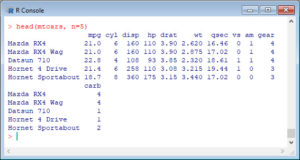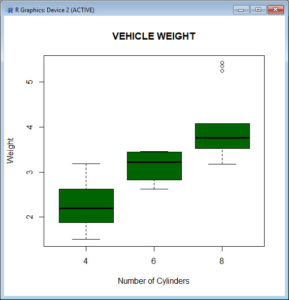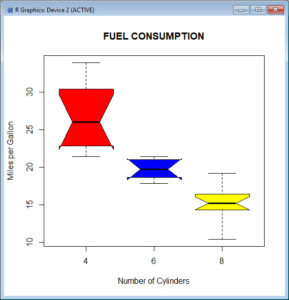In Part 13, let’s see how to create boxplots in R. Let’s create a simple boxplot using the boxplot() command, which is easy to use. First, we set up a vector of numbers and then we plot them.
Boxplots can be created for individual variables or for variables by group. The syntax is boxplot(x, data=), where x is a formula and data denotes the data frame providing the data. An example of a formula is: y ~ group, where you create a separate boxplot for each value of group.
Use varwidth=TRUE to make boxplot widths proportional to the square root of the sample sizes. Use horizontal=TRUE to reverse the axis orientation.
The Standard Boxplot does not indicate outliers. The Modified Boxplot highlights outliers. The Modified Boxplot is the default in R.
A <- c(3, 2, 5, 6, 4, 8, 1, 2, 3, 2, 4)
boxplot(A)R has some built-in datasets. One of them is mtcars dataset. Let’s look at the built-in dataset mtcars. Print the first five rows of this data frame.Now create a boxplot for vehicle weight for each type of car.
boxplot(wt~cyl, data=mtcars, main=toupper("Vehicle Weight"), font.main=3, cex.main=1.2, xlab="Number of Cylinders", ylab="Weight", font.lab=3, col="darkgreen")Let’s create a notched boxplot of miles per gallon for each type of car, with different colours for each box. Boxplots help us to to make a visual comparison across levels and check for equality of medians.
boxplot(mpg~cyl, data=mtcars, main=toupper("Fuel Consumption"), font.main=3, cex.main=1.2, col=c("red","blue", "yellow"), xlab="Number of Cylinders", ylab="Miles per Gallon", font.lab=3, notch=TRUE, range = 0)The notches do not overlap, so we have evidence for a difference in the medians.
NOTE: The range argument determines how far the plot whiskers extend out from the box. If range is positive, the whiskers extend to the datum that is no more than range times the interquartile range from the box. The argument range = 0 ensures that the whiskers extend to the data extremes. The argument horizontal=TRUE creates horizontal bars.
That wasn’t so hard! In Blog 14 we will look at further plotting techniques in R.
See you later!
David

Annex: R codes used

# Create a vector of numbers.
A <- c(3, 2, 5, 6, 4, 8, 1, 2, 3, 2, 4)
boxplot(A)

# Print the first five rows of mtcars data frame.